# Test: Basic & Electric Circuits

## 10 Questions MCQ Test Topicwise Question Bank for Electrical Engineering | Test: Basic & Electric Circuits

Description
Attempt Test: Basic & Electric Circuits | 10 questions in 30 minutes | Mock test for Electrical Engineering (EE) preparation | Free important questions MCQ to study Topicwise Question Bank for Electrical Engineering for Electrical Engineering (EE) Exam | Download free PDF with solutions
QUESTION: 1

### If the power supplied by the 20 V voltage source shown in figure below is 60 W then, the power supplied by the unknown circuit element (x) will be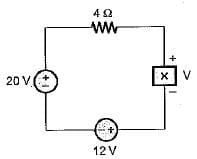Solution:

we know that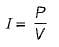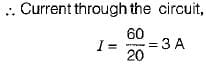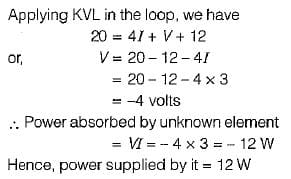QUESTION: 2

### In the network of figure shown below, I1 = 0.05 A, I2 = 0.1 A , R = 50 Ω. The unknown current source has strength of i, If 100 J of energy is delivered to I2 in 1 min, the magnitude of i will be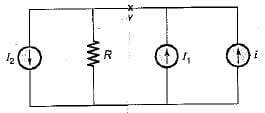Solution:

energy dissipated in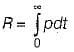where p=vi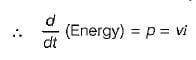Since power is constant, therefore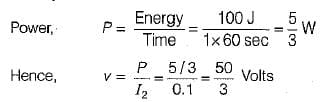Applying KCL at node v, we have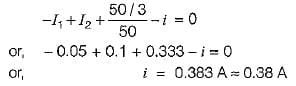QUESTION: 3

### The energy required to move 120 C charge through 3 V is

Solution:

W=QV=120 X 3 = 360J

QUESTION: 4

For a given voltage, four heating coils will produce maximum heat, when connected

Solution:

Maximum heat produced is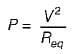for P to be maximum, Req should be minimum which is minimum of all four coils are connected in parallel.

QUESTION: 5

Given figure shows a plot of d.c. voltage applied to a resistor of 5 Ω for 15 seconds. The total energy consumed by the resistor is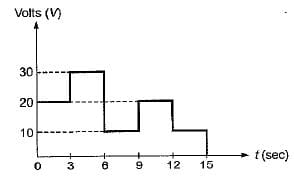Solution:

energy = power x time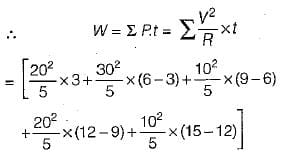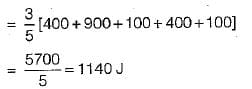Hence, the heat consumed by the resistor

= 1140 J

QUESTION: 6

The charge in a capacitor is given by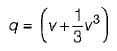if the voltage across this capacitor be v(t) = sin t, the current i(t) through the capacitor is

Solution:

The current through.the capacitor is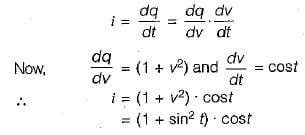QUESTION: 7

The unit of reactive power is

Solution:

Active power does do work, so it is the real axis. The unit for power is the watt (symbol: W). Apparent power is often expressed in volt-amperes (VA) since it is the product of rms voltage and rms current. The unit for reactive power is var, which stands for volt-ampere reactive.

QUESTION: 8

The unit of electrical energy is

Solution:
QUESTION: 9

The given signal is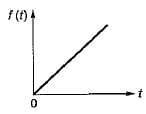Solution:

Given signal is a ramp signal f(t) = A(t), which is neither an energy signal nor a power signal.

QUESTION: 10

The two voltage sources connected in parallel as shown below, must satisfy the conditions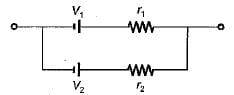Solution:Use Code STAYHOME200 and get INR 200 additional OFF Use Coupon Code